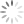Loading, please wait...# C# MCQ (Multiple Choice Questions and Answers)

May 29, 2020 C#, C#.Net, C# MCQs, 159483 Views
In this article we’ll go through various important questions of C#.Net .This would help students to test their knowledge, in a way that to what extent they are familiar with the topic. This article would even help students to brush up their knowledge.

Are you preparing for the next job interviews in C# .NET? If yes, trust me this post will help you also we'll suggest you check out a big collection for Programming Full Forms that may help you in your interview:

## List of Programming Full Forms

The questions are as follows along with the options given below them:

### 1. CLR is the .Net equivalent of _____.

1. Java Virtual machine
2. Common Language Runtime
3. Common Type System
4. Common Language Specification

Ans:  A

### 2. Abstract class contains _____.

1. Abstract methods
2. Non Abstract methods
3. Both
4. None

Ans:  C

1. private
2. public
3. protected
4. internal

Ans:  B

### 4. Which of the following statements is incorrect about delegate?

1. Delegates are reference types.
2. Delegates are object-oriented.
3. Delegates are type-safe.
4. Only one can be called using a delegate.

Ans : D

1. True
2. False
3. Maybe
4. Can’t say

Ans: A

### 6. Which of the following is incorrect about constructors?

1. Defining of constructors can be implicit or explicit.
2. The calling of constructors is explicit.
3. Implicit constructors can be parameterized or parameterless.
4. Explicit constructors can be parameterized or parameterless.

Ans: C

### 7. Reference is a ___.

1. Copy of class which leads to memory allocation.
2. Copy of class that is not initialized.
3. Pre-defined data type.
4. Copy of class creating by an existing instance.

Ans: D

### 8. The data members of a class by default are?

1. protected, public
2. private, public
3. private
4. public

Ans: C

### 9. What is the value returned by function compareTo( ) if the invoking string is less than the string compared?

1. Zero
2. A value of less than zero
3. A value greater than zero
4. None of the mentioned

Ans: B

### 10.  The correct way to overload +operator?

1. public sample operator +  (sample a, sample b)
2. public abstract operator +  (sample a, sample b)
3. public static operator +  (sample a, sample b)
4. all of the mentioned above

Ans: D

### 11. Select the two types of threads mentioned in the concept of multithreading?

1. Foreground
2. Background
3. Only foreground
4. Both foreground and background

Ans: D

### 12. Choose the wrong statement about properties used in C#.Net?

1. Each property consists of accessor as getting and set.
2. A property cannot be either read or write-only.
3. Properties can be used to store and retrieve values to and from the data members of a class.
4. Properties are like actual methods that work like data members.

Ans: A

### 13. If a class ‘demo’ had ‘add’ property with getting and set accessors, then which of the following statements will work correctly?

2. math m =  new math();
4. None of the mentioned

Ans: A

### 14. What will be the output of the following code snippet?

``````using System;
class sample
{
int i;
double k;
public sample (int ii, double kk)
{
i = ii;
k = kk;
double j = (i) + (k);
Console.WriteLine(j);
}
~sample()
{
double j = i - k;
Console.WriteLine(j);
}
}
class Program
{
static void Main(string[] args)
{
sample s = new sample(9, 2.5);
}
}``````
1. 0   0
2. 11.5   0
3. Compile-time error
4. 11.5 6.5

Ans : D

### 15. What will be the output of the following code snippet?

``````using System;
class program
{
static void Main(string[] args)
{
int x = 8;
int b = 16;
int c = 64;
x /= c /= b;
Console.WriteLine(x + " " + b+ " " +c);
}
}``````
1. 2  16   4
2. 4   8   16
3. 2   4    8
4. 8  16  64

Ans: A

1. Protected
2. Public
3. Private
4. Default

Ans:  C

### 17. The point at which an exception is thrown is called the _____.

1. Default point
2. Invoking point
3. Calling point
4. Throw point

Ans:  D

### 18. Which of the following statements are correct for C# language?

1. Every derived class does not define its own version of the virtual method.
2. By default, the access mode for all methods in C# is virtual.
3. If a derived class, does not define its own version of the virtual method, then the one present in the base class gets used.
4. All of the above.

Ans: C

### 19. What will be the output of the following code snippet?

``````using System;

class sample
{
public sample()
{
Console.WriteLine("constructor 1 called");
}
public sample(int x)
{
int p = 2;
int u;
u = p + x;
Console.WriteLine("constructor 2 called");
}
}
class Program
{
static void Main(string[] args)
{
sample s = new sample(4);
sample t = new sample();
}
}``````
1. constructor 1 called
constructor 2 called
2. constructor 2 called
constructor 1 called
3. constructor 2 called
constructor 2 called
4. error

Ans: B

1. this
2. static
3. base
4. extend

Ans: C